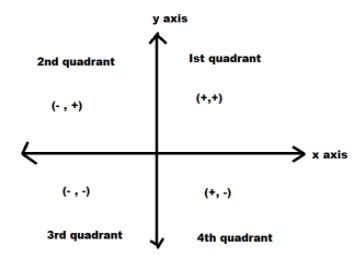QuestionAnswers

# State which quadrant or which axis do the following points lie1. $A{\text{ }}\left( { - 3,{\text{ }}2} \right)$2. $B{\text{ }}\left( {5,{\text{ }}3} \right)$3. $C{\text{ }}\left( {0,{\text{ }}4} \right)$4. $D{\text{ }}\left( { - 3,{\text{ }}0} \right)$Verified
129k+ views
Hint: Here we check for each of the given point :
*If the first coordinate i.e. the x coordinate as well as the second coordinate i.e. the y coordinate are positive then the point is in the first quadrant.
*If the first coordinate i.e. the x coordinate is negative and the second coordinate i.e. the y coordinate is positive then the point is in the second quadrant.
*If the first coordinate i.e. the x coordinate as well as the second coordinate i.e. the y coordinate are negative then the point is in the third quadrant.
*If the first coordinate i.e. the x coordinate is positive and the second coordinate i.e. the y coordinate is negative then the point is in the fourth quadrant.
*If the first coordinate i.e. the x coordinate is zero then the point lies on y axis
*If the second coordinate i.e. the y coordinate is zero then the point lies on x axisLet us consider point A
1. The given point is $A{\text{ }}\left( { - 3,{\text{ }}2} \right)$
The x coordinate is +5
The y coordinate is +3
Now as the first coordinate i.e. the x coordinate is negative and the second coordinate i.e. the y coordinate is positive.
Therefore the given point A lies in the second quadrant.

Now let us consider point B
2. The given point is $B{\text{ }}\left( {5,{\text{ }}3} \right)$
The x coordinate is -3
The y coordinate is +2
Now as the first coordinate i.e. the x coordinate as well as the second coordinate i.e. the y coordinate are positive.
Therefore the given point B lies in the first quadrant.

Now let us consider point C
3. The given point is $C{\text{ }}\left( {0,{\text{ }}4} \right)$
The x coordinate is 0
The y coordinate is +4
Now as the first coordinate i.e. the x coordinate is zero.
Therefore the given point C lies on y axis.

Now let us consider point D
4. The given point is $D{\text{ }}\left( { - 3,{\text{ }}0} \right)$
The x coordinate is -3
The y coordinate is 0
Now as the second coordinate i.e. the y coordinate is zero.
Therefore the given point D lies on the x axis.

Note: Students may make mistakes while checking the signs of the respective coordinates. So the signs of the coordinates should be checked carefully and then the points should be assigned quadrants according to the coordinates.